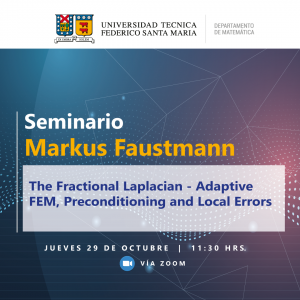# Seminario Markus Faustmann

Publicado el: 28 · Octubre · 2020

The Fractional Laplacian - Adaptive FEM, Preconditioning and Local Errors

Este jueves 29 de octubre a las 11:30 horas Markus Faustmann, investigador postdoctoral del Institute for Analysis and Scientific Computing, Vienna University of Technology, dictará la charla denominada ” The Fractional Laplacian – Adaptive FEM, Preconditioning and Local Errors”.

Enlace de Zoom

ID de reunión: 851 9906 6300

Código de acceso: 980735

Resumen:

In this talk, we consider fractional powers of the Laplacian (−∆)s, s∈(0,1). As there are various ways of defining such operators, we present a brief introduction to some of the different definitions and then mainly focus on the so-called integral fractional Laplacian given as a singular integral. For the integral fractional Laplacian, we analyze various new aspects of numerical approximation by the finite element method (FEM).

We start with analyzing an adaptive FEM. As solutions to fractional PDEs on bounded domains tend to behave singularly across the whole boundary of the domain, it is natural to refine the finite element mesh locally towards the boundary. An adaptive algorithm of the form Solve – Estimate – Mark – Refine chooses the region for refinement automatically according to some computed a-posteriori error indicators that estimate the element wise errors. We present novel refinement indicators of residual type that are valid for all fractional parameters s and prove optimal convergence rates of the adaptive FEM steered by these error indicators.

A drawback of the adaptive FEM is that the condition number of the system matrices gets worse as one refines the mesh. In order to counteract that, we propose an additive Schwarz preconditioner of BPX-type for the fractional Laplacian on locally refined meshes that leads to uniformly bounded condition numbers.

Finally, we analyze the local convergence behavior of the FEM for the integral fractional Laplacian on quasi-uniform meshes. We present sharp a-priori estimates in the H1-norm, where the local error is bounded by the local best-approximation error and a global error in a weak norm. Using duality arguments, we obtain sharp rates of convergence locally.

Descarga el PDF